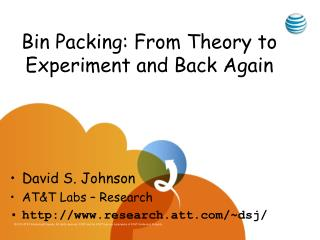DownloadDownload PresentationBin Packing: From Theory to Experiment and Back Again

Bin Packing: From Theory to Experiment and Back Again

Download PresentationBin Packing: From Theory to Experiment and Back Again

- - - - - - - - - - - - - - - - - - - - - - - - - - - E N D - - - - - - - - - - - - - - - - - - - - - - - - - - -
Presentation Transcript

1. Bin Packing: From Theory to Experiment and Back Again • David S. Johnson • AT&T Labs – Research • http://www.research.att.com/~dsj/

2. Applications • Packing commercials into station breaks • Packing files onto floppy disks • Packing MP3 songs onto CDs • Packing IP packets into frames, SONET time slots, etc. • Packing telemetry data into fixed size packets Standard Drawback: Bin Packing is NP-complete

3. OUTLINE • Worst-Case Performance • Average-Case Performance • Classical Models • Experiments  Theory • Discrete Distributions • Theory  Experiments  Theory

4. First Fit (FF):Put each item in the first bin with enough space Best Fit (BF):Put each item in the bin that will hold it with the least space left over First Fit Decreasing, Best Fit Decreasing (FFD,BFD): Start by reordering the items by non-increasing size.

5. Worst-Case Bounds • Theorem [Ullman, 1971]. For all lists L, BF(L), FF(L) ≤ (17/10)OPT(L) + 3. • Theorem [Johnson, 1973]. For all lists L, BFD(L), FFD(L) ≤ (11/9)OPT(L) + 4. (Note 1: 11/9 = 1.222222…) (Note 2: These bounds are asymptotically tight.)

6. Lower Bounds: FF and BF ½ -  ½ -  ½ -  ½ +  ½ +  OPT: N bins FF, BF: N/2 bins + N bins = 1.5 OPT

7. Lower Bounds: FF and BF 1/6 - 2 1/6 - 2 1/6 - 2 1/6 - 2 1/6 - 2 1/6 - 2 1/6 - 2 1/3 + 1/3 + 1/3 + ½ +  1/2 + OPT: N bins FF, BF: N/6 bins + N/2 bins + N bins = 5/3 OPT

8. Lower Bounds: FF and BF 1/43 + , 1/1806 + , etc. 1/3 + 1/7 +  FF, BF = N(1 + 1/2 + 1/6 + 1/42 + 1/1805 + … )  (1.691..) OPT 1/2 + OPT: N bins

9. “Improving” on FF and BF

10. “Improving” on FFD and BFD

11. Average-Case Performance

12. Progress?

13. Progress:Faster Computers  Bigger Instances

14. Definitions

15. Definitions, Continued

16. Theorems for U[0,1]

17. Distributions U[0,u] Item sizes uniformly distributed in the interval (0,u], 0 < u < 1

18. Average Waste for BF under U(0,u]

19. Measured Average Waste for BF under U(0,.01]

20. Conjecture

21. FFD on U(0,u] u = .6 FFD(L) – s(L) u = .5 u = .4 N = Experimental Results from [Bentley, Johnson, Leighton, McGeoch, 1983]

22. FFD on U(0,u], u  0.5

23. FFD on U(0,u], u  0.5

24. FFD on U(0,u], 0.5  u  1

25. OUTLINE  • Worst-Case Performance • Average-Case Performance • Classical Models • Experiments  Theory • Discrete Distributions • Theory  Experiments  Theory  

26. Discrete Distributions

27. Courcoubetis-Weber

28. y z (0,2,1) (1,0,2) (2,1,1) x (0,0,0)

29. Courcoubetis-Weber Theorem

30. A Flow-Based Linear Program

31. Theorem [Csirik et al. 2000] Note: The LP’s for (1) and (3) are both of size polynomial in B, not log(B), and hence “pseudo-polynomial”

32. U{6,8} U{12,16} U{3,4} U(0,¾] 1 2/3 1/3 0.00 0.25 0.50 0.75 1.00 Discrete Uniform Distributions

33. Theorem [Coffman et al. 1997] (Results analogous to those for the corresponding U(0,u])

34. Experimental Results for Best Fit0 ≤ u ≤ 1, 1 ≤ j ≤ k = 51 Averages of 25 trials for each distribution, N = 2,048,000

35. Average Waste under Best Fit(Experimental values for N = 100,000,000 and 200,000,000) [KRS, 1996] Holds for all j = k-2 [GJSW, 1993]

36. Theorem [Kenyon & Mitzenmacher, 2000]

37. Average wBF(L)/s(L) for U{j,85}

38. Average wBFD(L)/s(L) for U{j,85}

39. Averages on the Same Scale

40. The Discrete Distribution U{6,13}

41. ¾β 6 β/24 3 2 3 3 3 3 4 6 2 5 5 2 4 2 β/6 β/2 β/2 2 4 2 β/2 β/2 β/3 β/8 β/24 “Fluid Algorithm” Analysis: U{6,13} Size = 6 5 4 3 2 1 Amount = ββββββ Bin Type = Amount =

42. Expected Waste

43. Theorem[Coffman, Johnson, McGeoch, Shor, & Weber, 1994-2008]

44. Waste as a Function of j and k (mod 6)